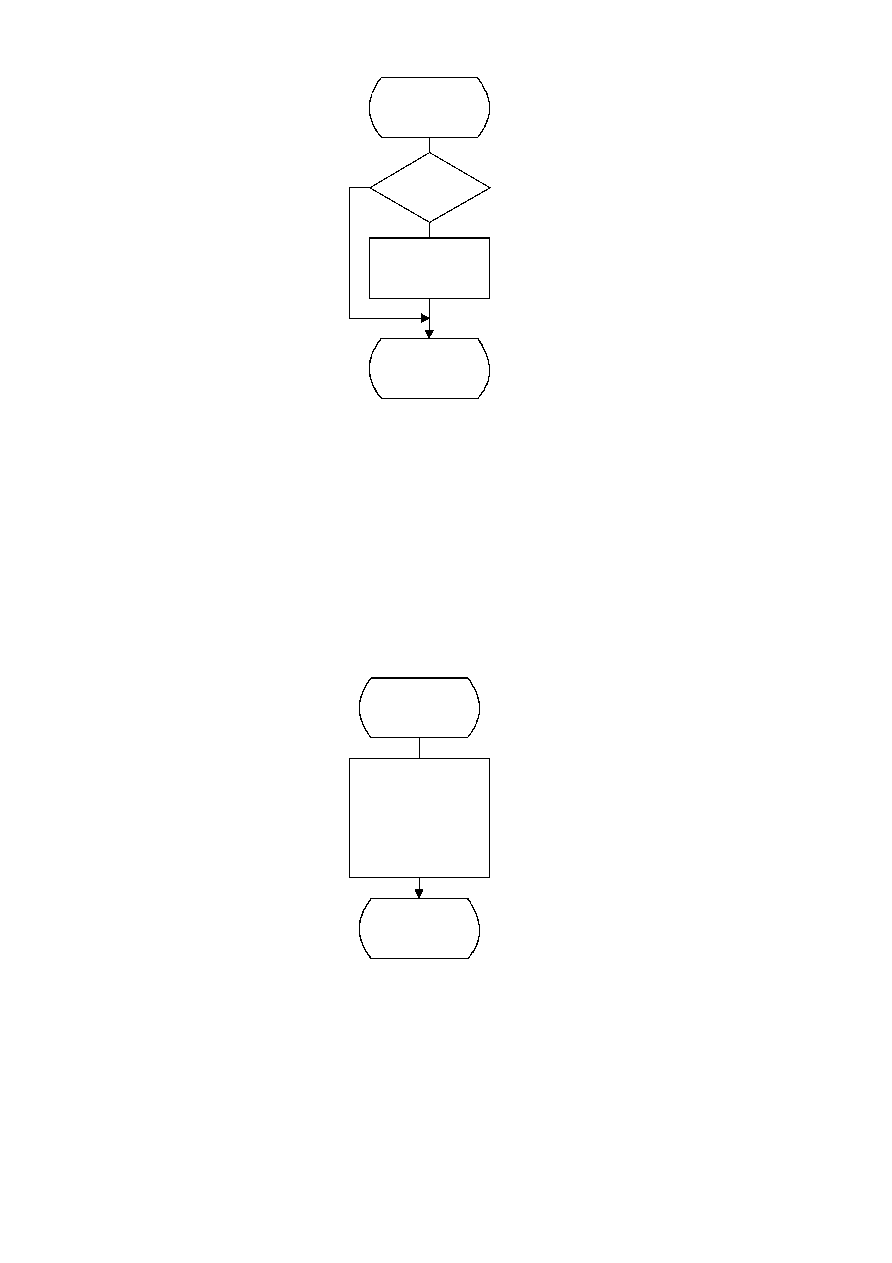ISO/IEC 10918-1 : 1993(E)
TISO1110-93/d049
Stuff_0
No
Yes
Done
BP = BP + 1
B = 0
B = X'FF'
?
Figure D.11 ­ Stuff_0 procedure for encoder
Figure D.11 [D49], = 10 cm = 391.%
D.1.7
Initialization of the encoder
The Initenc procedure is used to start the arithmetic coder. The basic steps are shown in Figure D.12.
TISO1120-93/d050
Initenc
Done
Initialize statistics areas
ST = 0
A = X'10000'
A =
(see Note below)
C = 0
CT = 11
BP = BPST ­ 1
Figure D.12 ­ Initialization of the encoder
Figure D.12 [D50], = 9 cm = 352.%
66
CCITT Rec. T.81 (1992 E)